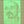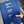0%
0%

# Measuring the Effective Permittivity of PCBs

Find out how you can measure the effective permittivity (a.k.a. dielectric constant) of your PCB substrate.

Similar projects worth following
1.9k views
Knowing the effective permittivity εᵣ, also known as the dielectric constant, can be important when working with PCBs at RF. For example, impedance of a microstrip line and the resonant frequency of a PCB antenna depend on this parameter. A good PCB fabricator will ensure that the impedance of a transmission line is correct, but cheap fabricators used by people like myself don't do this (and so they're cheaper of course!). A widely used method for measuring this value involves fabricating a ring resonator structure, which is what I'm doing with this project.

The cheap PCB fabricators don't have any sort of guarantees on impedance, which is part of the reason they are inexpensive. My thought is that if a specific impedance is required, you could fabricate this calibration structure, measure it, and use it to tweak your line width (or other geometry). Then order the PCB form the same supplier quickly and hope that the εᵣ is still the same. Or, you can fabricate it along with your PCB just out of curiosity as a way to check the εᵣ.

### gerbers_resonator_2.zip

Gerber files for a ~915 MHz resonator on a 1.6 mm thick PCB (2 layers).

Zip Archive - 8.83 kB - 05/08/2018 at 02:30

### resonator_calculations.m

A MATLAB script that calculates relative permittivity using resonant frequencies measured in the lab.

- 2.16 kB - 05/08/2018 at 02:29

• 1 × Resonator PCB
• 2 × SMA connector
• 1 × Vector Network Analyzer Lab Instrument

• ### Measurement Results

Ville Tiukuvaara05/28/2018 at 01:24 1 comment

Here is an assembled resonator. Nice to only have to solder on a couple SMAs rather than a whole bunch of components and be able to get to measuring right away.

Based on my calculations, for a 1.6 mm PCB with 1 oz. copper (0.0175 mm layer), the substrate should be 1.565 mm thick. When I measured with a calliper, the thickness came in at 1.52 mm, which includes the bottom copper pour. So I used a thickness of 1.51 mm in the calculations.

The VNA I used to measure the resonators went up to 20 GHz, but the shape of the graph started to go wonky after about 13 GHz. I suppose some other phenomenon starts to show up after that. This still gives me 15 points at which to calculate the dielectric constant.

I updated the MATLAB script to take an array of the frequencies where there are minimums of S21 (the resonant frequencies) and plot of graph of the relative permittivity over frequency. Here is what I got:The relative permittivity of the FR-4 over frequency, calculated using the measured resonant frequencies.

The trend of decreasing εᵣ over frequency is consistent with the graph towards the end of this article. Also, the permittivity is greater than 4.4 that I have been nominally using in my calculations.

• ### The Physics of Ring Resonators

Ville Tiukuvaara05/08/2018 at 02:28 3 comments

Transmission lines like the microstrip are an essential ingredient in RF PCBs. The trace width required for a given characteristic impedance Z₀ can easily be calculated using online tools or KiCAD's calculator utility. One of the important parameters is the relative permittivity εᵣ of the substrate, also known as the dielectric constant. Companies that have cash often use expensive substrate materials that have a precise εᵣ over frequency and between lots, which means that they can be confident that the characteristic impedance will be consistent. Good PCB fabricators will also ensure that transmission line impedances are correct, but this is not the case with the cheap fabricators. Hobbyists usually rely on FR-4, which might have a relative permittivity anywhere between 4.35 and 4.8 (usually nominally taken as 4.4). That's a ±10% change, which would change a 50 Ω trace on a 1.6 mm PCB to 48 Ω at εᵣ = 4.8. That doesn't sound like much, and maybe it's OK. However, sometimes it is more critical.

What do you do if you need to know the relative permittivity of your substrate? There are many papers written on the topic, but I'm going to give the skinny on one of these, which involves measuring a ring resonator and some pretty straight-forward math. The details are laid out here, and you can also calculate the loss tangent, but I'm not going there so read the paper if you're interested!

# Ring Resonators

The ring resonator, shown below, is on a 10 cm x 10 cm PCB, making it cheap to fab (\$2 at JLCPCB). The structure is a ring that is separated by a small gap to traces that lead to the SMA connectors. On the bottom there is a solid ground plane, so the traces are microstrip transmission lines.

To calculate the resonances of the ring, it can be thought of as being a straight line rather than a circle, which is appropriate if the width w of the line is much less than the radius r; this is called the "straight-line approximation". This article recommends that r/w > 7. At the resonant frequencies, there will be a standing wave, which mathematically requires that

Here, v is the phase velocity, c₀ is the speed of light, and εᵣ,eff is the effective relative permittivity. So we can measure the resonances and easily calculate εᵣ,eff. This in turn can be used to calculate the actual εᵣ of the substrate (e.g. FR-4) through a series of equations from various papers, nicely summarized here. These equations also capture the frequency-dependence. I have written a MATLAB program (in the files section of this project) that implements these calculations.

I was interested in measuring at 915 MHz in the ISM band, for which I calculated a radius of ~294 mm using a trace width of 1.5 mm and a 1.6 mm thick PCB. Unfortunately the PCB I sent to fab had a 293 mm radius that I found using some other calculations (which I believe are less accurate based on the simulations below). I made the microstrip lines from the SMA connectors 50 Ω since I will be measuring this with a VNA.

# Simulation in HFSS

The coupling of the ring across the gaps can affect the resonance, so I was keen to simulate the resonator. I set up two models in HFSS, one with SMA connectors and one where the transmission lines were excited directly. To model the SMA connectors I followed this video on YouTube. As expected, the first resonance happened close to 915 MHz.The magnitude of the electric field at the first resonance (close to 915 MHz). The actual copper traces are hidden to make it easier to see the electric field.

The second resonance is to be expected around 1830 MHz, which...

Share

## Discussions

Justin Kenny wrote 04/23/2020 at 07:59 point

Excellent work. Can you use this method to measure the loss tangent too?

Are you sure? yes | no

## Similar Projects

Project Owner Contributor

### The Rise and Fall of PulsesTed Yapo

Project Owner Contributor

### TDR sampler/pulse generator "pod" (TDR-G3)MS-BOSS

# Does this project spark your interest?

Become a member to follow this project and never miss any updates